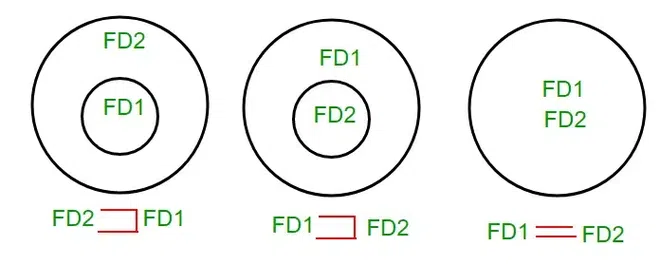# Equivalence of Functional Dependencies

For understanding the equivalence of Functional Dependencies Sets (FD sets), the basic idea about Attribute Closure is given in this article Given a Relation with different FD sets for that relation, we have to find out whether one FD set is a subset of another or both are equal.

## How To Find the Relationship Between Two Functional Dependency Sets?

Let FD1 and FD2 be two FD sets for a relation R.

1. If all FDs of FD1 can be derived from FDs present in FD2, we can say that FD2 ⊃ FD1.
2. If all FDs of FD2 can be derived from FDs present in FD1, we can say that FD1 ⊃ FD2.
3. If 1 and 2 both are true, FD1=FD2.

All these three cases can be shown using the Venn diagram:Equivalence of Functional Dependency

## Why We Need to Compare Functional Dependencies?

Suppose in the designing process we convert the ER diagram to a relational model and this task is given to two different engineers. Now those two engineers give two different sets of functional dependencies. So, being an administrator we need to ensure that we must have a good set of Functional Dependencies. To ensure this we require to study the equivalence of Functional Dependencies.

• It can help to identify redundant functional dependencies, which can be eliminated to reduce data redundancy and improve database performance.
• It can help to optimize database design by identifying equivalent sets of functional dependencies that can be used interchangeably.
• It can ensure data consistency by identifying all possible combinations of attributes that can exist in the database.

• The process of determining the equivalence of functional dependencies can be computationally expensive, especially for large datasets.
• The process may require testing multiple candidate sets of functional dependencies, which can be time-consuming and complex.
• The equivalence of functional dependencies may not always accurately reflect the semantic meaning of data, and may not always reflect the true relationships between data elements.

## Sample Questions

Q.1 Let us take an example to show the relationship between two FD sets. A relation R(A,B,C,D) having two FD sets FD1 = {A->B, B->C, AB->D} and FD2 = {A->B, B->C, A->C, A->D}

Step 1: Checking whether all FDs of FD1 are present in FD2

• A->B in set FD1 is present in set FD2.
• B->C in set FD1 is also present in set FD2.
• AB->D is present in set FD1 but not directly in FD2 but we will check whether we can derive it or not. For set FD2, (AB)+ = {A, B, C, D}. It means that AB can functionally determine A, B, C, and D. So AB->D will also hold in set FD2.

As all FDs in set FD1 also hold in set FD2, FD2 ⊃ FD1 is true.

Step 2: Checking whether all FDs of FD2 are present in FD1

• A->B in set FD2 is present in set FD1.
• B->C in set FD2 is also present in set FD1.
• A->C is present in FD2 but not directly in FD1 but we will check whether we can derive it or not. For set FD1, (A)+ = {A, B, C, D}. It means that A can functionally determine A, B, C, and D. SO A->C will also hold in set FD1.
• A->D is present in FD2 but not directly in FD1 but we will check whether we can derive it or not. For set FD1, (A)+ = {A, B, C, D}. It means that A can functionally determine A, B, C, and D. SO A->D will also hold in set FD1.

As all FDs in set FD2 also hold in set FD1, FD1 ⊃ FD2 is true.

Step 3: As FD2 ⊃ FD1 and FD1 ⊃ FD2 both are true FD2 =FD1 is true. These two FD sets are semantically equivalent.

Q.2 Let us take another example to show the relationship between two FD sets. A relation R2(A,B,C,D) having two FD sets FD1 = {A->B, B->C,A->C} and FD2 = {A->B, B->C, A->D}

Step 1:  Checking whether all FDs of FD1 are present in FD2

• A->B in set FD1 is present in set FD2.
• B->C in set FD1 is also present in set FD2.
• A->C is present in FD1 but not directly in FD2 but we will check whether we can derive it or not. For set FD2, (A)+ = {A, B, C, D}. It means that A can functionally determine A, B, C, and D. SO A->C will also hold in set FD2.

As all FDs in set FD1 also hold in set FD2, FD2 ⊃ FD1 is true.

Step 2:  Checking whether all FDs of FD2 are present in FD1

• A->B in set FD2 is present in set FD1.,
• B->C in set FD2 is also present in set FD1.
• A->D is present in FD2 but not directly in FD1 but we will check whether we can derive it or not. For set FD1, (A)+ = {A,B,C}. It means that A can’t functionally determine D.
• So A->D will not hold in FD1.

As all FDs in set FD2 do not hold in set FD1, FD2 ⊄ FD1

Step 3: In this case, FD2 ⊃ FD1 and FD2 ⊄ FD1, these two FD sets are not semantically equivalent.

### 1. What is Functional Dependency?

Functional Dependency is basically the relationship between the attributes of a table that are inter dependent on each other.